simulation of three clusters combining to create a galaxy Time = 120 Two clusters of 200 N Matter particles each are orbiting a third of 100 normal matter particles.  The orbiting clusters are in a circular orbit at a radius of 20 units. Note ·        The simulation assumes a fixed anti-gravity matter field comprising a central anti-gravity matter hole radius 2 units and outside that an anti-gravity matter vortex such that orbital velocity of normal matter particles remains fairly constant over a wide range of radii.  (see Simulation Introduction for further explanation) ·        Normal matter particles feel a small drag force (proportional to the square of their velocity) except when they are following close behind another normal matter particle. ·        In all images the left hand image is the top view and the right hand image is the side view.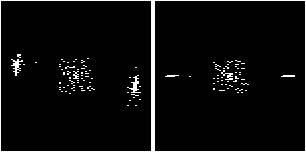Time = 898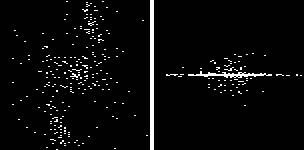Time = 1102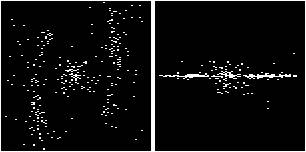Time = 1269Time =1583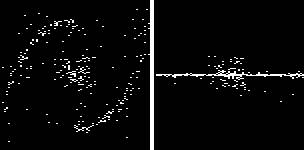Time =1912   Notes Compare with NGC 1300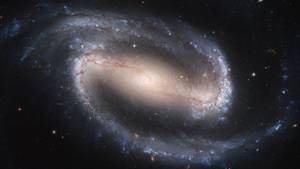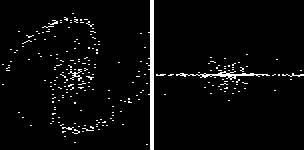Time = 2153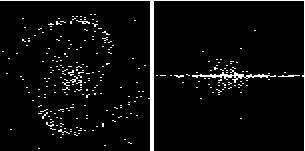Time = 2350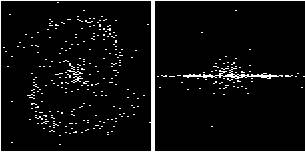Time = 2651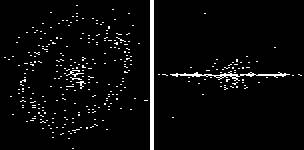Time = 2808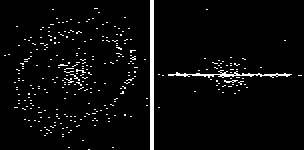Time = 2925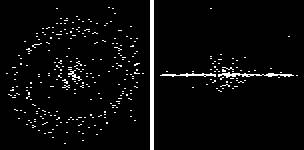Time = 3084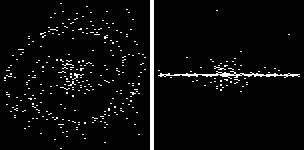Time = 3201   Notes And again similar to NGC 1300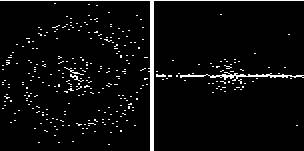Time = 3317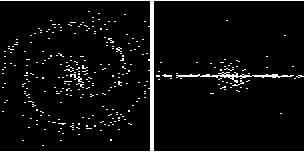Time = 3743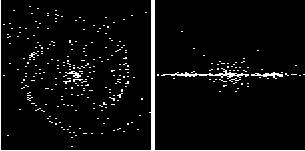Time = 3938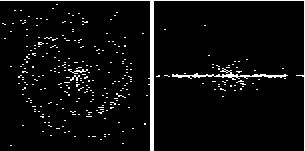Time = 4168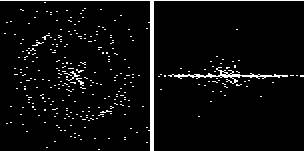Time = 4303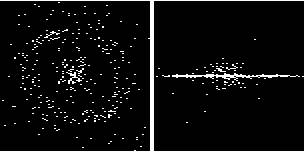Time = 4534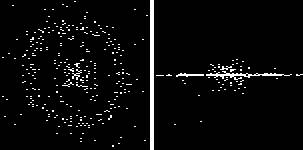Time = 5150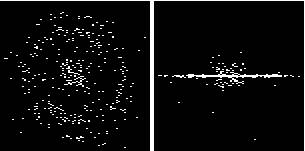Time = 5256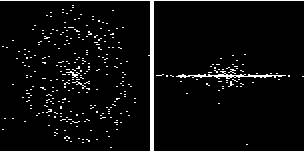Time = 5396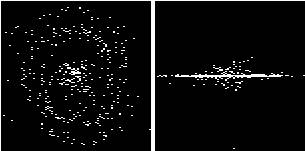Time = 5564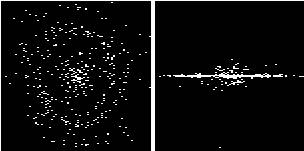Time = 5792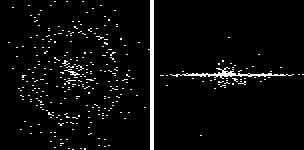Time = 6027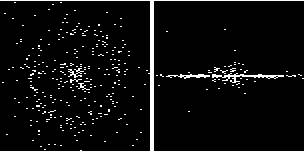Time = 7199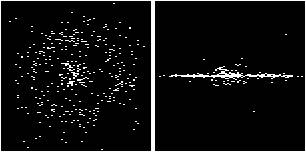Time = 8004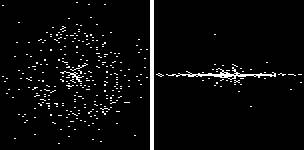Time = 10010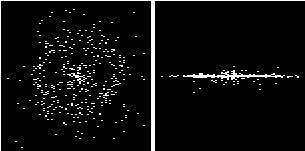Time = 11001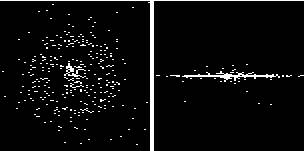Time = 12000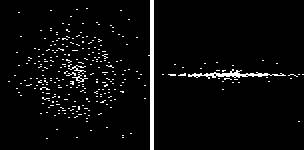Time = 14000Time = 16005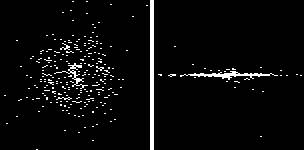Time = 18024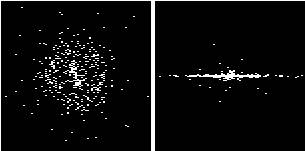Time = 20007   Notes The movement of particles in the disk is approximately circular.  Orbital velocity and speed are approximately the same. Particles in the core are moving mostly along a radius.  Their orbital velocity is low all the time but their speed increases as they fall towards and through the centre of the core, and reduces to nothing as they approach the edge of the core.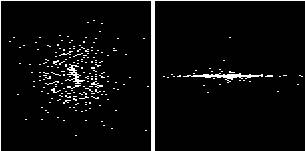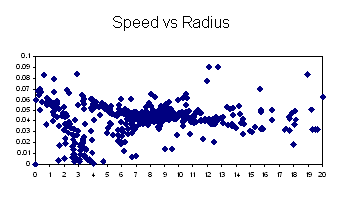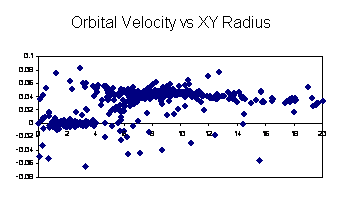Time = 40003   Notes The cluster still consists of two distinct populations of particles.  The inner one is moving mainly along a radius and the outer one is orbiting. Compare the simulated orbital velocity profile with the Andromeda orbital velocity profile.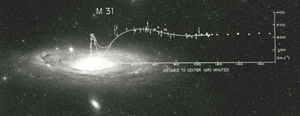The inner edge of the simulation’s orbital velocity hump is at a radius of about 2 units, which is also the radius of the core’s AGM Boundary.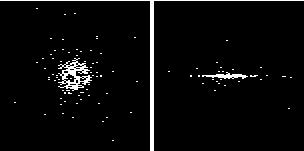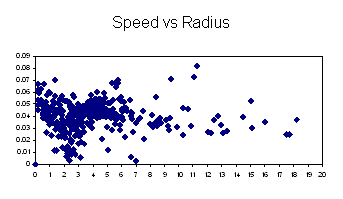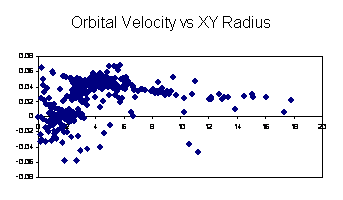Time = 60211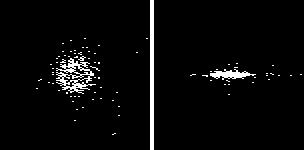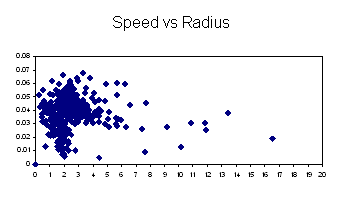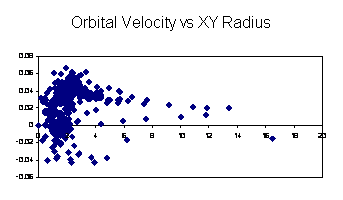Copyright © Tim E Simmons 2006, 2009